# Capacitor in Series and Parallel:

Generally we knows capacitor is nothing but two charging plates separated by insulating medium. and capacitor used for storage device and also used for filter purpose. there are different types of capacitors used in the electrical field for different applications.  Here we are going to see about how to add capacitor in series and parallel connection.

## Capacitor in Series

Let us consider C is the capacitor and we will going to add C1,C2,C3 … Cn capacitors in series and we will going to apply voltage V in across these capacitors . let see the below diagram,the voltage drop across that capacitor is v1,v2,v3…vn .if Q is the charge transferred to the capacitor then voltage drop V equal to, these capacitors, then,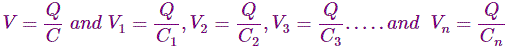so the charging of the capacitor can be added like this below,
Now, equation (i) can be written as,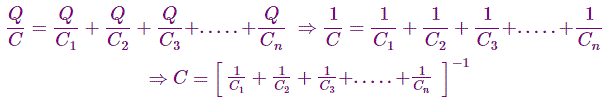## Capacitors in Parallel

Now we are going to connect C1,C2,C3…Cn number of capacitor in parallel connections. the combination of capacitor in parallel is connected to voltage source V. the equivalent capacitance of capacitor C1,C2,C3 …. Cn are C.

The blow diagram will show you capacitor in parallel connection.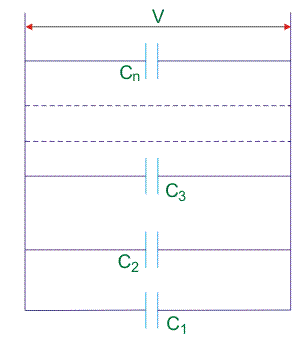Let us consider the capacitance are connected in parallel connection. so the current flow in the each capacitor will be same. the total charge of the parallel combinations depend on each capacitors. the voltage drop across the each capacitance will be same.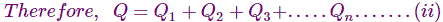Where,Q1, Q2, Q3,…….Qn are the charge of capacitor C1, C2, C3….. Cn respectively.Now equation (2) can be written as,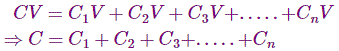Now we can see that adding of capacitance in series is 1/C1+1/C2+….1/Cn but adding of capacitance in parallel is just summation of capacitor like C1+C2+C3……..Cn.

## One thought on “Capacitors in Series and Parallel”

1.StevenNen says:

Hello